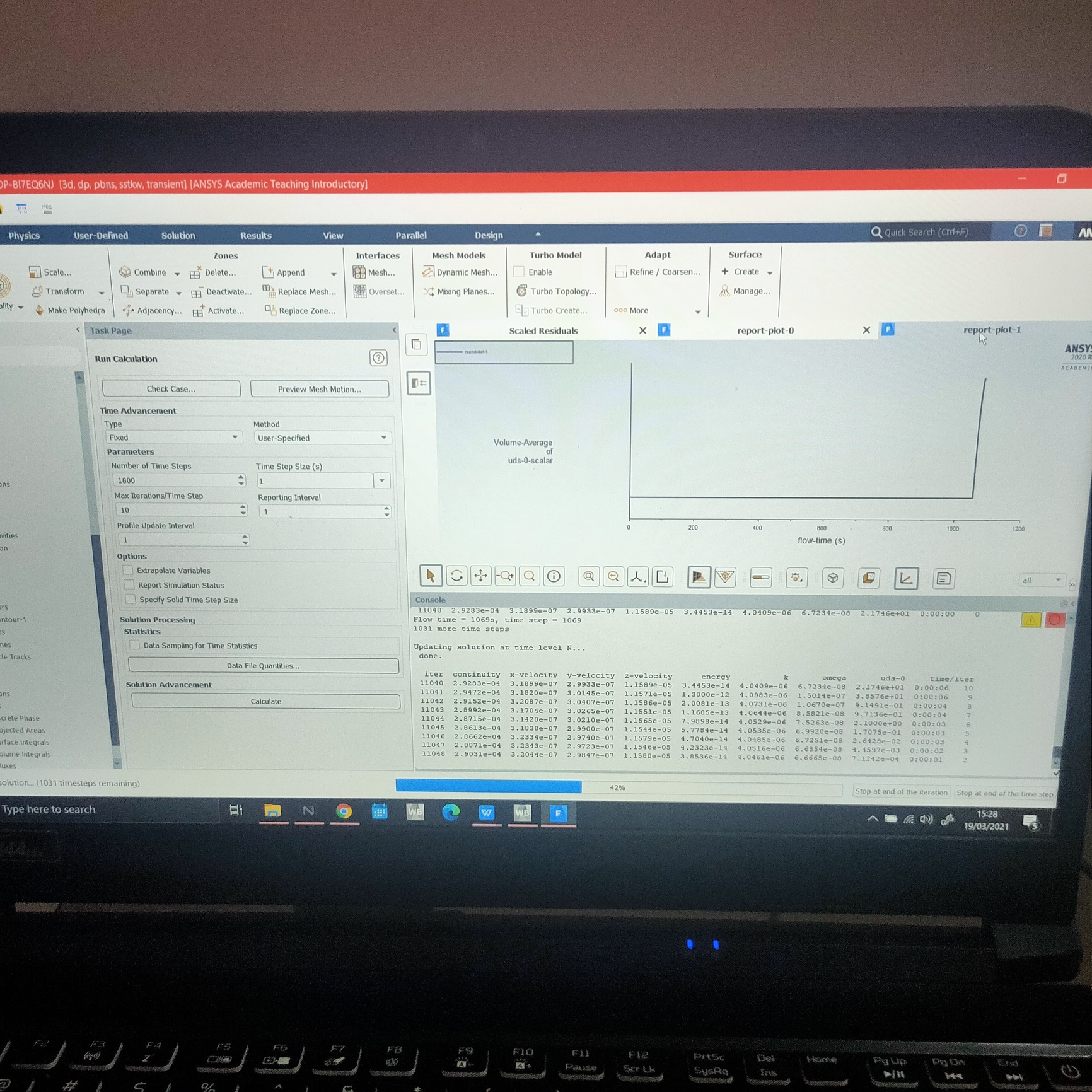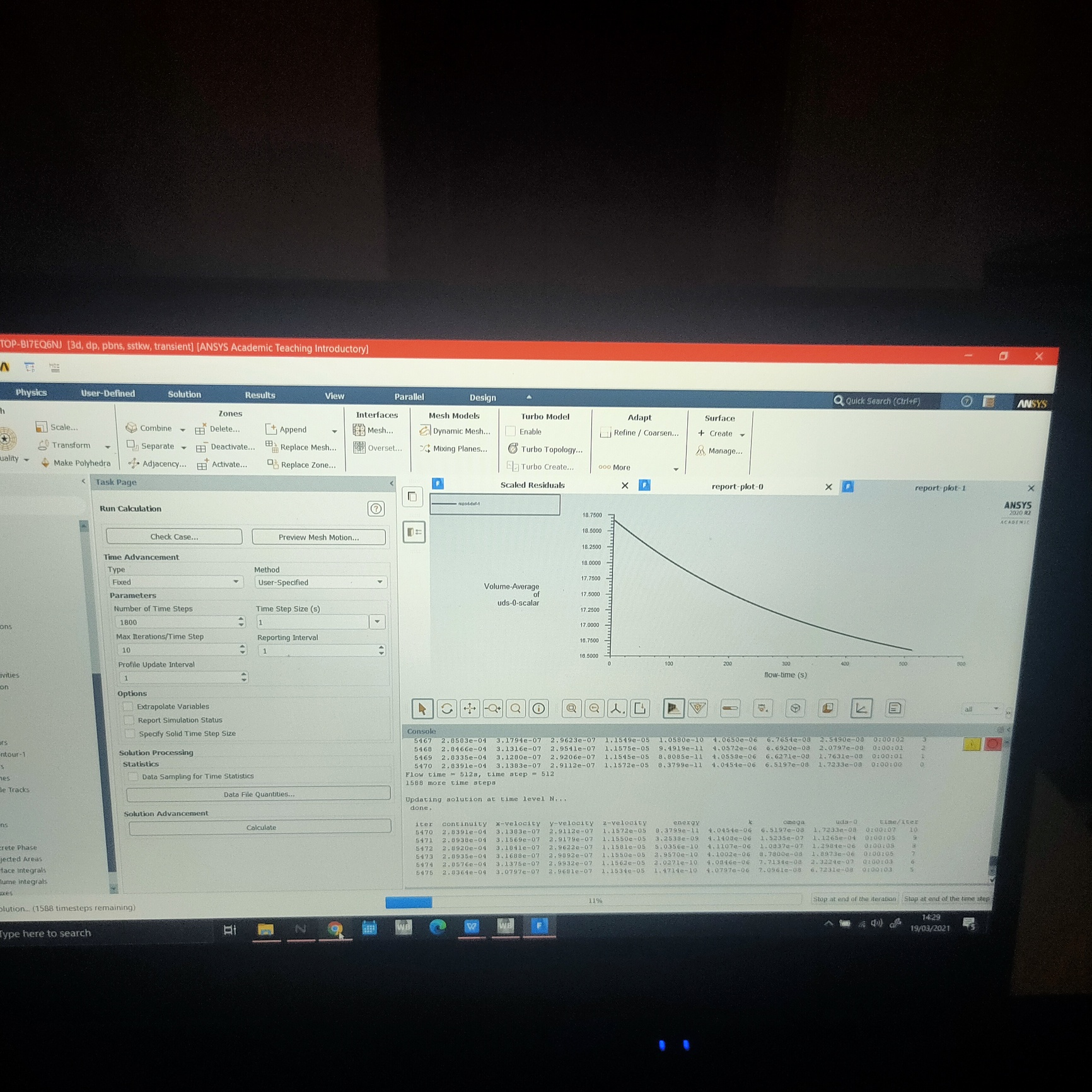## Fluids

•Alvin
Subscriber
Hi, I am currently modeling heat and mass (moisture) transfer using UDS scalar as moisture inside a kernel. I used the equation hm*(Ms-Ma) at the air-kernel interface, where hm is convective mass transfer coefficient, Ms is surface moisture (also Scalar 0) and Ma is air moisture (UDM 0). For some time, the moisture of the kernel is dropping as it is being dried by hot air, but at some point, the exponential drying curve changes into a different and unrealistic graph. What seems to be the problem? And how can I fix this?nn
•DrAmine
Ansys Employee
Quite hard to deliver here a guidance without knowing to certain extend: which models are involved and how are you defining the mass transfer via UDF. Also please describe the problem.n
•Alvin
SubscriberThank you for replying nI'm using Ansys Student 2020 R2, I used expressions to create the mass transfer equation instead of using UDF. I used a converged steady state solution before modelling transient heat and mass transfer. The simulation solves for continuity, energy, turbulence (bcoz of SST k-omega model) and UDS. I monitored the simulation by plotting the vol average of Scalar 0 (moisture content) over time. As you can see in the pictures, it started fine, showing exponential trend, then after some time, the trend changes into an absurd one. What could be the reason behind this??n
•Rob
Ansys Employee
Plot the expression and see if there are any oddities in it's behaviour at certain temperature, pressure or mass fraction conditions. n
•Alvin
Subscriber
Hi thanks for replying.nwhen you say plot the expression, do you mean the named expression? or the variable such as scalar 0??n
•Rob
Ansys Employee
Any and all expressions that are used to set properties or boundaries. It may be something else is causing the problem, but it's often a badly defined curve/profile or sudden step in values that then causes the solver to diverge. n